## RS Aggarwal Class 7 Solutions Chapter 20 Mensuration Ex 20F

These Solutions are part of RS Aggarwal Solutions Class 7. Here we have given RS Aggarwal Solutions Class 7 Chapter 20 Mensuration Ex 20F.

Other Exercises

Question 1.
Solution:
(i) Radius of the circle (r) = 21 cm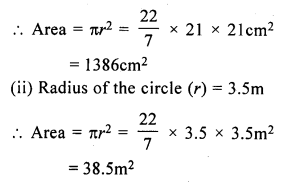Question 2.
Solution:
(i) Diameter of the circle = 28 cm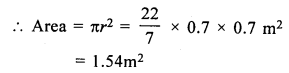Question 3.
Solution:
Circumference of a circle = 264 cmQuestion 4.
Solution:
Circumference of the circle (c) = 35.2 mQuestion 5.
Solution:
Area of the circle = 616 cm²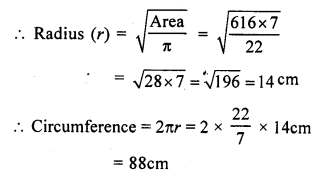Question 6.
Solution:
Area of a circle = 1386 m²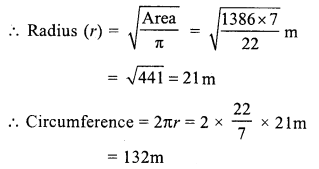Question 7.
Solution:
Ratio in the radii of two circles = 4 : 5
Let radius of first circle (r1) = 4x
and radius of the second circle (r2) = 5x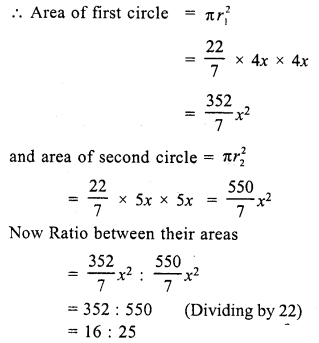Question 8.
Solution:
Length of rope (r) = 21 m
Area of the circle = πr² = $$\frac { 22 }{ 7 }$$ x 21 x 21 m² = 1386 m²
The horse will graze on 1386 m² area

Question 9.
Solution:
Area of a square made of a wire = 121 cm²
Side = √Area = √121 = 11 m
Perimeter of wire = 4 x side = 4 x 11 = 44 cm
Circumference of circular wire = 44 cm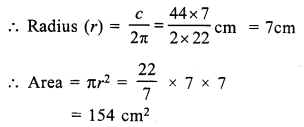Question 10.
Solution:
Radius of circular wire = 28 cm
Circumference = 2πr = 2 x $$\frac { 22 }{ 7 }$$ x 28 cm = 176 cm
Perimeter of the square formed by this wire = 176 cm
Side (a) = $$\frac { 176 }{ 4 }$$ = 44 cm .
Area of square so formed = a² = (44)² cm² = 1936 cm²

Question 11.
Solution:
Length of rectangular sheet (l) = 34 cm
and breadth (b) = 24 cm
Area = l x b = 34 x 24 cm² = 816 cm²
Diameter of one button = 3.5 cm
Radius (r) = $$\frac { 3.5 }{ 2 }$$ cm
and area of one button = πr²Area of 64 buttons = 9.625 x 64 cm² =616 cm²
Area of remaining sheet = 816 – 616 = 200 cm²

Question 12.
Solution:
Length of ground (l) = 90 m
and breadth (b) = 32 m
Area = l x b = 90 x 32 m² = 2880 m²
Radius of circular tank (r) = 14 m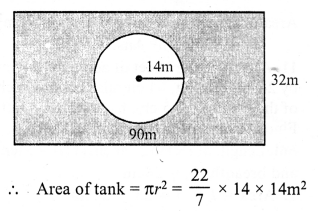= 616 m²
Area of remaining portion = 2880 – 616 = 2264 m²
Rate of turfing = Rs. 50 per sq.m.
Total cost = Rs. 50 x 2264 = Rs. 113200

Question 13.
Solution:
Each side of square = 14 cm
Area of square = a² = 14 x 14 = 196 cm²
Radius of each circle at each corner of square = $$\frac { 14 }{ 2 }$$ = 7 cm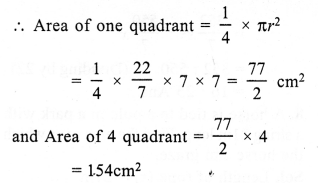Area of shaded portion = Area of square – area of 4 quadrants
= 196 – 154 = 42 cm²

Question 14.
Solution:
Length of field = 60 m
Length of rope = 14 m
Area covered by the horse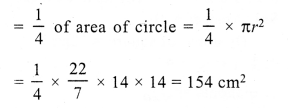Question 15.
Solution:
Diameter of largest circle (outer circle) = 21 cm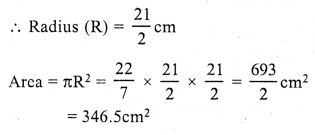Area of shaded portion = 346.5 – (154 + 38.5) = (346.5 – 192.5) = 154 cm²

Question 16.
Solution:
Length of plot (l) = 8m